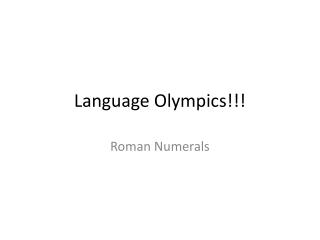Download PresentationLanguage Olympics!!!

Loading in 2 Seconds...

# Language Olympics!!! - PowerPoint PPT Presentation

Language Olympics!!!. Roman Numerals. Olympic Event. This week’s Olympics will be an individual event in Roman Numerals. Winners will receive extra credit on the Summer Reading Notebooks! Woot ! Students will get five minutes to review the Roman Numeral section of their Language Olympics.I am the owner, or an agent authorized to act on behalf of the owner, of the copyrighted work described.
Download Presentation## Language Olympics!!!

An Image/Link below is provided (as is) to download presentation

Download Policy: Content on the Website is provided to you AS IS for your information and personal use and may not be sold / licensed / shared on other websites without getting consent from its author.While downloading, if for some reason you are not able to download a presentation, the publisher may have deleted the file from their server.

- - - - - - - - - - - - - - - - - - - - - - - - - - E N D - - - - - - - - - - - - - - - - - - - - - - - - - -
Presentation Transcript
1. Language Olympics!!! Roman Numerals

2. Olympic Event • This week’s Olympics will be an individual event in Roman Numerals. • Winners will receive extra credit on the Summer Reading Notebooks! Woot! • Students will get five minutes to review the Roman Numeral section of their Language Olympics. • Don’t have your Language Olympics with you? • Oops. Too bad. Sit silently and don’t disturb others while they study.

3. Everybody Stand Up! • Put notes away. No notes allowed during this event!!! • We will go around the room. • Each student will get a Roman Numeral. • Mrs. Mehrens, Dr. Woodstellman, and Senora Flores will call on you when it is your turn. • You will have TEN seconds to translate into Arabic numerals (1, 2, 3, 4, 5…) • If you get it right, you stay standing. • If you get it wrong, you sit down. • Last ones standing get extra credit… • 1st place = 3 points • 2nd place = 2 points • 3rd place = 1 point

4. V Answer = 5

5. III Answer = 3

6. VII Answer = 7

7. X Answer = 10

8. XI Answer = 11

9. I Answer = 1

10. XX Answer = 20

11. L Answer = 50

12. LV Answer = 55

13. LXX Answer = 70

14. LXXV Answer = 75

15. C Answer = 100

16. CIII Answer = 103

17. CVII Answer = 107

18. CXXV Answer = 125

19. CXVII Answer = 117

20. CC Answer = 200

21. CCCXXXIII Answer = 333

22. D Answer = 500

23. DCC Answer = 700

24. DCCCXXV Answer = 825

25. M Answer = 1000

26. MMXII Answer = 2012

27. MMMDCLI Answer = 3651

28. IV Answer = 4

29. IX Answer = 9

30. XIV Answer = 14

31. XIX Answer = 19

32. XXIV Answer = 24

33. XXIX Answer = 29

34. XL Answer = 40

35. XC Answer = 90

36. CD Answer = 400

37. CM Answer = 900

38. XLIV Answer = 44

39. XCIX Answer = 99

40. XV Answer = 15

41. DCX Answer = 610

42. XLVII Answer = 47

43. MCCXXX Answer = 1230

44. XXI Answer = 21

45. LXII Answer = 62

46. CCCXXVII Answer = 327

47. CCLXIII Answer = 263

48. DXXI Answer = 521

49. XXVI Answer = 26

50. MCMLXXXV Answer = 1985